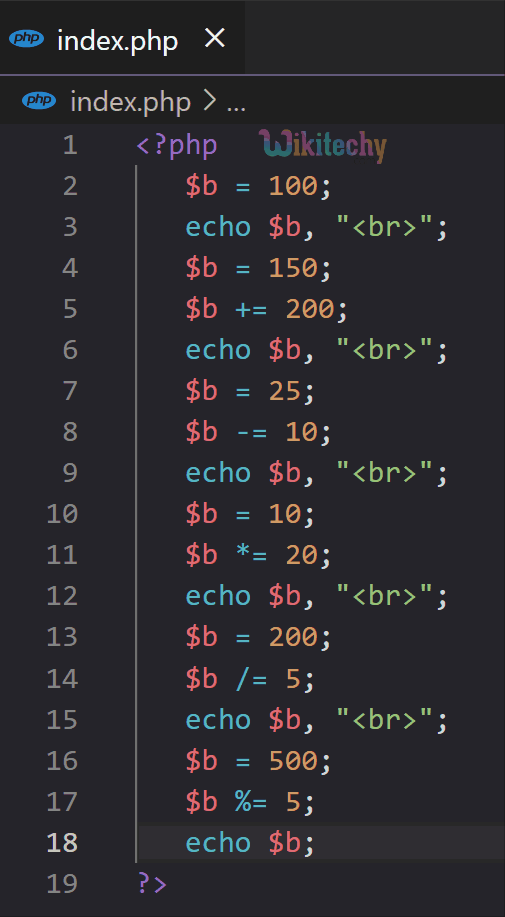# PHP Assignment Operators - Assignment Operators in PHP

## PHP Assignment Operators

• PHP assignment operators are used to assign a value to a variable. They are the most common type of operator used in PHP.
• Examples include the "=" operator, which assigns a value to a variable, and the "+=" operator, which adds a value to the variable and assigns the result.
Operator Syntax Operation
Assign (=) \$a = \$b Operand on the left obtains the value of the operand on the right
Add then Assign (+=) \$a += \$b Simple Addition same as \$a = \$a + \$b
Subtract then Assign (-=) \$a -= \$b Simple subtraction same as \$a = \$a – \$b
Multiply then Assign (*=) \$a *= \$b Simple product same as \$x = \$a * \$b
Divide then Assign (quotient - /=) \$a /= \$b Simple division same as \$x = \$a / \$b
Divide then Assign (remainder - %=) \$a %= \$b Simple division same as \$x = \$a % \$b